## 97. Examples using MPI_SCATTER, MPI_SCATTERVUp: Scatter Next: Gather-to-all Previous: Scatter

The examples in this section use intracommunicators.
Example The reverse of Example Examples using MPI_GATHER, MPI_GATHERV . Scatter sets of 100 ints from the root to each process in the group. See Figure 9 .

```    MPI_Comm comm;
int gsize,*sendbuf;
int root, rbuf;
...
MPI_Comm_size( comm, &gsize);
sendbuf = (int *)malloc(gsize*100*sizeof(int));
...
MPI_Scatter( sendbuf, 100, MPI_INT, rbuf, 100, MPI_INT, root, comm);
```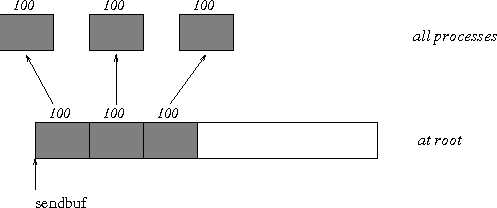Example The reverse of Example Examples using MPI_GATHER, MPI_GATHERV . The root process scatters sets of 100 ints to the other processes, but the sets of 100 are stride ints apart in the sending buffer. Requires use of MPI_SCATTERV. Assume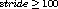. See Figure 10 .

```    MPI_Comm comm;
int gsize,*sendbuf;
int root, rbuf, i, *displs, *scounts;

...

MPI_Comm_size( comm, &gsize);
sendbuf = (int *)malloc(gsize*stride*sizeof(int));
...
displs = (int *)malloc(gsize*sizeof(int));
scounts = (int *)malloc(gsize*sizeof(int));
for (i=0; i<gsize; ++i) {
displs[i] = i*stride;
scounts[i] = 100;
}
MPI_Scatterv( sendbuf, scounts, displs, MPI_INT, rbuf, 100, MPI_INT,
root, comm);
```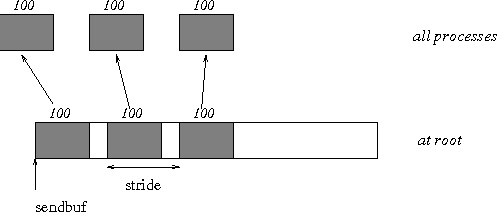Example The reverse of Example Examples using MPI_GATHER, MPI_GATHERV . We have a varying stride between blocks at sending (root) side, at the receiving side we receive into the i-th column of a 100×150 C array. See Figure 11 .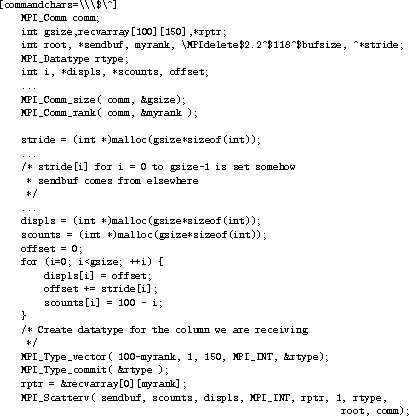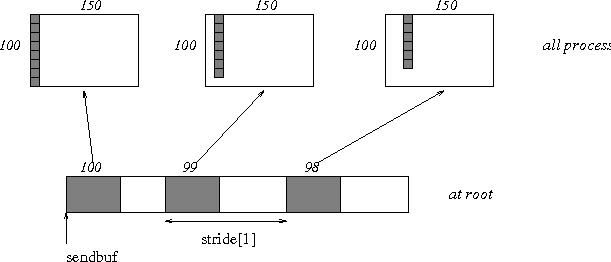Up: Scatter Next: Gather-to-all Previous: Scatter